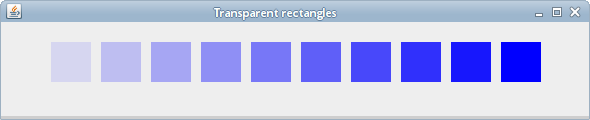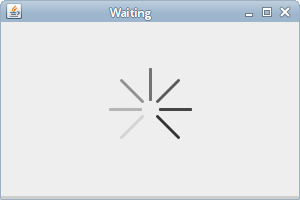# Transparency

In this part of the Java 2D, we talk about transparency. We provide some basic definitions and several interesting transparency effects.

## Transparency explained

Transparency is the quality of being able to see through a material. The easiest way to understand transparency is to imagine a piece of glass or water. Technically, the rays of light can go through the glass and this way we can see objects behind the glass.

In computer graphics, we can achieve transparency effects using alpha compositing. Alpha compositing is the process of combining an image with a background to create the appearance of partial transparency. The composition process uses an alpha channel. Alpha channel is an 8-bit layer in a graphics file format that is used for expressing translucency (transparency). The extra eight bits per pixel serves as a mask and represents 256 levels of translucency.
(answers.com, wikipedia.org)

The `AlphaComposite` class is used to work with transparency in Java 2D. It implements the basic alpha compositing rules for combining source and destination pixels to achieve blending and transparency effects with graphics and images. To create an `AlphaComposite`, we provide two values: the rule designator and the alpha value. The rule specifies how we combine source and destination pixels. Most often it is `AlphaComposite.SRC_OVER`. The alpha value can range from 0.0f (completely transparent) to 1.0f (completely opaque).

## Transparent rectangles

The first example draws ten rectangles with different levels of transparency.

TransparentRectanglesEx.java
```package com.zetcode;

import java.awt.AlphaComposite;
import java.awt.Color;
import java.awt.EventQueue;
import java.awt.Graphics;
import java.awt.Graphics2D;
import javax.swing.JFrame;
import javax.swing.JPanel;

class Surface extends JPanel {

private void doDrawing(Graphics g) {

Graphics2D g2d = (Graphics2D) g.create();

g2d.setPaint(Color.blue);

for (int i = 1; i <= 10; i++) {

float alpha = i * 0.1f;
AlphaComposite alcom = AlphaComposite.getInstance(
AlphaComposite.SRC_OVER, alpha);
g2d.setComposite(alcom);
g2d.fillRect(50 * i, 20, 40, 40);
}

g2d.dispose();
}

@Override
public void paintComponent(Graphics g) {

super.paintComponent(g);
doDrawing(g);
}
}

public class TransparentRectanglesEx extends JFrame {

public TransparentRectanglesEx() {

initUI();
}

private void initUI() {

add(new Surface());

setTitle("Transparent rectangles");
setSize(590, 120);
setLocationRelativeTo(null);
setDefaultCloseOperation(JFrame.EXIT_ON_CLOSE);
}

public static void main(String[] args) {

EventQueue.invokeLater(new Runnable() {
@Override
public void run() {

TransparentRectanglesEx ex = new TransparentRectanglesEx();
ex.setVisible(true);
}
});
}
}
```

In our example we draw 10 blue rectangles with various levels of transparency applied.

```float alpha = i * 0.1f;
```

The alpha value dynamically changes in the for loop.

```AlphaComposite alcom = AlphaComposite.getInstance(
AlphaComposite.SRC_OVER, alpha);
```

The `AlphaComposite.getInstance()` method creates an `AlphaComposite` object with the specified rule and the constant alpha to multiply with the alpha of the source.

```g2d.setComposite(alcom);
```

The `setComposite()` method sets the composite attribute for the `Graphics2D` object.Figure: Transparent rectangles

## Fade out demo

In the next example, we will fade out an image. The image will gradually get more transparent until it is completely invisible.

FadeOutEx.java
```package com.zetcode;

import java.awt.AlphaComposite;
import java.awt.Dimension;
import java.awt.EventQueue;
import java.awt.Graphics;
import java.awt.Graphics2D;
import java.awt.Image;
import java.awt.event.ActionEvent;
import java.awt.event.ActionListener;
import javax.swing.ImageIcon;
import javax.swing.JFrame;
import javax.swing.JPanel;
import javax.swing.Timer;

class Surface extends JPanel
implements ActionListener {

private Image img;
private Timer timer;
private float alpha = 1f;

private final int DELAY = 40;
private final int INITIAL_DELAY = 500;

public Surface() {

loadImage();
setSurfaceSize();
initTimer();
}

private void loadImage() {

img = new ImageIcon("mushrooms.jpg").getImage();
}

private void setSurfaceSize() {

int h = img.getHeight(this);
int w = img.getWidth(this);
setPreferredSize(new Dimension(w, h));
}

private void initTimer() {

timer = new Timer(DELAY, this);
timer.setInitialDelay(INITIAL_DELAY);
timer.start();
}

private void doDrawing(Graphics g) {

Graphics2D g2d = (Graphics2D) g.create();

AlphaComposite acomp = AlphaComposite.getInstance(
AlphaComposite.SRC_OVER, alpha);
g2d.setComposite(acomp);
g2d.drawImage(img, 0, 0, null);

g2d.dispose();
}

@Override
public void paintComponent(Graphics g) {

super.paintComponent(g);
doDrawing(g);
}

private void step() {

alpha += -0.01f;

if (alpha <= 0) {

alpha = 0;
timer.stop();
}
}

@Override
public void actionPerformed(ActionEvent e) {

step();
repaint();
}
}

public class FadeOutEx extends JFrame {

public FadeOutEx() {

initUI();
}

private void initUI() {

add(new Surface());

pack();

setTitle("Fade out");
setLocationRelativeTo(null);
setDefaultCloseOperation(JFrame.EXIT_ON_CLOSE);
}

public static void main(String[] args) {

EventQueue.invokeLater(new Runnable() {
@Override
public void run() {

FadeOutEx ex = new FadeOutEx();
ex.setVisible(true);
}
});
}
}
```

With `AlphaComposite`, we gradually fade out an image on the panel.

```private void setSurfaceSize() {

int h = img.getHeight(this);
int w = img.getWidth(this);
setPreferredSize(new Dimension(w, h));
}
```

The `setSurfaceSize()` method finds out the size of the image and sets a preferred size for the panel. The preferred size with the combination of the `pack()` method will display the window just large enough to show the whole image.

```private void initTimer() {

timer = new Timer(DELAY, this);
timer.setInitialDelay(INITIAL_DELAY);
timer.start();
}
```

The `initTimer()` method starts a timer. The timer fires action events after the specified initial delay. Successive action events are generated after between-event delay. In reaction to an action event, we will change the alpha value and repaint the panel.

```AlphaComposite acomp = AlphaComposite.getInstance(
AlphaComposite.SRC_OVER, alpha);
g2d.setComposite(acomp);
g2d.drawImage(img, 0, 0, null);
```

This code draws an image with increasing levels of transparency on the panel.

```private void step() {

alpha += -0.01f;

if (alpha <= 0) {

alpha = 0;
timer.stop();
}
}
```

The `step()` method represents a fade out cycle. The `alpha` is gradually decreased. Note that the alpha value must not be negative. When it reaches zero, the timer is stopped.

```repaint();
```

The `repaint()` method repaints the component. It invokes the `paint()` method of the panel component, which in turn calls the `paintComponent()` method.

## Waiting demo

In this example, we use transparency effect to create a waiting demo. We draw 8 lines that gradually fade out creating an illusion that a line is moving. Such effects are often used to inform users that a lengthy task is going on behind the scenes. For instance, when streaming video over the Internet.

WaitingEx.java
```package com.zetcode;

import java.awt.AlphaComposite;
import java.awt.BasicStroke;
import java.awt.EventQueue;
import java.awt.Graphics;
import java.awt.Graphics2D;
import java.awt.RenderingHints;
import java.awt.event.ActionEvent;
import java.awt.event.ActionListener;
import javax.swing.JFrame;
import javax.swing.JPanel;
import javax.swing.Timer;

class Surface extends JPanel
implements ActionListener {

private Timer timer;
private int count;
private final int INITIAL_DELAY = 200;
private final int DELAY = 80;
private final int NUMBER_OF_LINES = 8;
private final int STROKE_WIDTH = 3;

private final double[][] trs = {
{0.0, 0.15, 0.30, 0.5, 0.65, 0.80, 0.9, 1.0},
{1.0, 0.0, 0.15, 0.30, 0.5, 0.65, 0.8, 0.9},
{0.9, 1.0, 0.0, 0.15, 0.3, 0.5, 0.65, 0.8},
{0.8, 0.9, 1.0, 0.0, 0.15, 0.3, 0.5, 0.65},
{0.65, 0.8, 0.9, 1.0, 0.0, 0.15, 0.3, 0.5},
{0.5, 0.65, 0.8, 0.9, 1.0, 0.0, 0.15, 0.3},
{0.3, 0.5, 0.65, 0.8, 0.9, 1.0, 0.0, 0.15},
{0.15, 0.3, 0.5, 0.65, 0.8, 0.9, 1.0, 0.0}
};

public Surface() {

initTimer();
}

private void initTimer() {

timer = new Timer(DELAY, this);
timer.setInitialDelay(INITIAL_DELAY);
timer.start();
}

private void doDrawing(Graphics g) {

Graphics2D g2d = (Graphics2D) g.create();

g2d.setRenderingHint(RenderingHints.KEY_ANTIALIASING,
RenderingHints.VALUE_ANTIALIAS_ON);
g2d.setRenderingHint(RenderingHints.KEY_RENDERING,
RenderingHints.VALUE_RENDER_QUALITY);

int width = getWidth();
int height = getHeight();

g2d.setStroke(new BasicStroke(STROKE_WIDTH, BasicStroke.CAP_ROUND,
BasicStroke.JOIN_ROUND));
g2d.translate(width / 2, height / 2);

for (int i = 0; i < NUMBER_OF_LINES; i++) {

float alpha = (float) trs[count % NUMBER_OF_LINES][i];
AlphaComposite acomp = AlphaComposite.getInstance(
AlphaComposite.SRC_OVER, alpha);
g2d.setComposite(acomp);

g2d.rotate(Math.PI / 4f);
g2d.drawLine(0, -10, 0, -40);
}

g2d.dispose();
}

@Override
public void paintComponent(Graphics g) {

super.paintComponent(g);
doDrawing(g);
}

@Override
public void actionPerformed(ActionEvent e) {

repaint();
count++;
}
}

public class WaitingEx extends JFrame {

public WaitingEx() {

initUI();
}

private void initUI() {

add(new Surface());

setTitle("Waiting");
setSize(300, 200);
setLocationRelativeTo(null);
setDefaultCloseOperation(JFrame.EXIT_ON_CLOSE);
}

public static void main(String[] args) {

EventQueue.invokeLater(new Runnable() {
@Override
public void run() {

WaitingEx ex = new WaitingEx();
ex.setVisible(true);
}
});
}
}
```

We draw eight lines with eight different alpha values.

```private final double[][] trs = {
...
};
```

This is a two dimensional array of transparency values used in this demo. There are 8 rows, each for one state. Each of the 8 lines will continuously use these values.

```g2d.setStroke(new BasicStroke(STROKE_WIDTH, BasicStroke.CAP_ROUND,
BasicStroke.JOIN_ROUND));
```

We make the lines a bit thicker so that they are better visible. We draw the lines with rounded caps.

```g2d.rotate(Math.PI/4f);
g2d.drawLine(0, -10, 0, -40);
```

This code draws each of the eight lines. The `rotate()` method is used to rotate the lines alongside a circle.Figure: Waiting

In this part of the Java 2D tutorial, we have talked about transparency.

ZetCode last modified April 15, 2015 © 2007 - 2019 Jan Bodnar Follow on Facebook